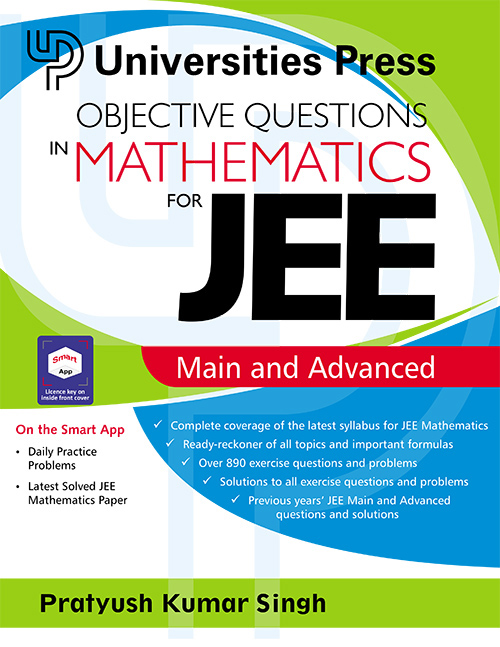## Objective Questions in Mathematics for JEE Main and Advanced

#### Price: 475.00

This book has been designed to provide students with intensive as well as extensive practice of the questions and problems they will encounter in the JEE Mathematics exams. The aim of this book is to improve and enhance the aptitude, proficiency and problem-solving ability of students in Mathematics to enable them to crown their endeavours with success.

Salient features:

• Key concepts at the beginning of every chapter to summarise the important principles
• Key equations and formulas presented for every topic
• Detailed, step-by-step solutions to all questions and problems
• Lavish use of figures to illustrate concepts and problems
• Appendices with previous years’ JEE Main and Advanced solved questions
• Android app with practice problems and solutions to the latest JEE Mathematics paper
• Video lectures available at: www.universitiespress.com/jeemaths

#### Contributors (Author(s), Editor(s), Translator(s), Illustrator(s) etc.)

Pratyush Kumar Singh obtained his B.Tech. in Civil Engineering from IIT Kanpur. He is the founder of an IIT coaching centre in Ranchi, has been teaching Mathematics and Physics for more than 25 years and has also authored several books on these subjects. His dedication and knowledge have helped more than 5000 students achieve their dreams.

Preface
Acknowledgements

PART I: ALGEBRA
Chapter 1: Theory of Equations
Chapter 3: Sequences and Series
Chapter 4: Binomial Theorem
Chapter 5: Complex Numbers
Chapter 6: Permutations and Combinations
Chapter 7: Probability
Chapter 8: Matrices
Chapter 9: Vector Algebra

PART II: CALCULUS
Chapter 10: Algebraic Functions
Chapter 11: Trigonometric Functions
Chapter 12: Trigonometric Equations
Chapter 13: Solution of Triangles
Chapter 14: Inverse Trigonometric Functions
Chapter 15: Exponential and Log Functions
Chapter 16: Limits and Continuity
Chapter 17: Investigating Functions
Chapter 18: Differentiation
Chapter 19: Application of Derivatives
Chapter 20: Indefinite Integration
Chapter 21: Definite Integration
Chapter 22: Differentiation of an Integral
Chapter 23: Area Between Curves
Chapter 24: Sum of a Series and Definite Integral
Chapter 25: Differential Equations

PART III: COORDINATE GEOMETRY
Chapter 26: Straight Line
Chapter 27: Circle
Chapter 28: Parabola
Chapter 29: Ellipse
Chapter 30: Hyperbola
Chapter 31: 3D Geometry

Appendix I: Previous Years’ JEE Main Questions and Solutions
Appendix II: Previous Years’ JEE Advanced Questions and Solutions

`